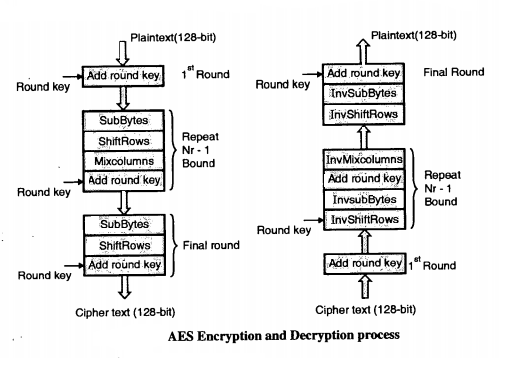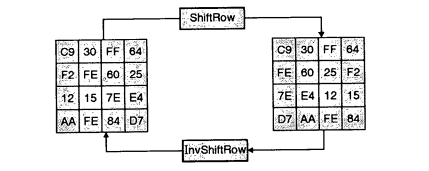# Advance Encryption Standard (AES) In Cryptography – The Ultimate Guide !!

The Advanced Encryption Standard (AES Algorithm) is a Symmetric-key cryptographic algorithm published by National Institute for Standards and Technology (NIST) in December 2001. The algorithm was proposed by Rijndael the reason also called Rijndael encryption algorithm. Advance encryption standard is a replacement of data encryption standard. So in this post, we will explore the process of AES (Advanced Encryption Standard) in detail .

## What Is AES (Advance Encryption Standard) In Cryptography ?

The AES algorithm is a subset of the Rijndael algorithm and is a block cipher, meaning that it operates on an input block of data of a known size and outputs a block of data that is the same size.

An input key is also required as input to the AES algorithm. It allows the data length (plain text size) of 128, 192 and 256 hits, and supporting three different key lengths, 128, 192, and 256 hits.

The AES algorithm is a symmetric key algorithm which means the same key is used to both encrypt and decrypt a message. Also, the ciphertext produced by the AES algorithm is the same size as the plain text message.

AES consists of multiple rounds for processing different key bits like 10 rounds for processing 128 – bit keys, 12 rounds for processing 192 – bit keys, and 14 rounds for processing 256 – hit keys.

## How Is The AES Encryption And Decryption Process Takes Place ?

The plaintext given is divided into 128 – bit block as consisting of a 4 x 4 matrix of bytes. Therefore, the first four bytes of a 128 – bit input block occupies the first column in the 4×4 matrix of bytes.

The next four bytes occupy the second column, and so on. AES operates on a 4 x 4 column-major order matrix of bytes, called as state array. AES also has the notion of a word. A word consists of four bytes that are 32 bits .

The number of rounds are 10, is for the case when the encryption key is 128 bit long. (As mentioned earlier, the number of rounds is 12 when the key is 192 bits and 14 when the key is 256.)

Before any round-based processing for encryption can begin each byte of the state (plaintext) is combined with the round key using bitwise XOR operation. Nr stands for a number of rounds.

AES divide plaintext into 16 byte (128-bit) blocks, and treats each block as a 4 x 4 State array . It then performs four operations in each round consists of several processing steps like the substitution step, a row-wise permutation step, a column-wise mixing step, and the addition of the round key.

Except for the last round in each case, all other rounds are identical. Final Round doesn’t have (MixColumns) it includes only SubBytes, ShiftRows and AddRoundKey .The process of transforming the ciphertext back into the original plaintext using the same encryption key is called as decryption process of AES, during the decryption process the set of rounds are reversed.

#### What Are The Detailed Steps In Advance Encryption Standard (AES) ?

For encryption, each round consists of the following four steps :

1.  Sub Bytes
2. Shift Rows
3. Mix Columns and

The SubByte step/Substitute byte In AES :

SubByte() consists of the replacement of each byte using a fixed S-box lookup table to achieve non-linearity into the 4 x 4 state array (16 byte). It performs roughly the same function as the S – BOX in DES.

It operates on each byte in the state and performs a non-linear substitution in the Galios Filed GF (2^8) field, which is what makes AES a non-linear cryptographic system.

State transformation will be done using SubBytes techniques and if apply reverse called as InvSubBytes transformation which will create original values. For every same two-byte value the resulting transformation is also same. It also shows that the InvSubBytes transformation creates the original one.

Note that if the two bytes have the same values, their transformation is also the same. The corresponding substitution step used during decryption is called InvSubBytes.

ShiftRows In AES Encryption :

The output of the SubByte transformation is input to the ShiftRows transformation which consists of rotation of each byte of the state array in the order of a row of data matrix (rotation of row byte positions are done in this step).Each byte of the first row remains unchanged. Each byte of the second row is rotated over one byte to the left position. Similarly, the third and fourth rows are also rotated left by two and three position .

MixColumns In AES Encryption :

Mix Columns performs an operation on the state array obtained from ShiftRows column-by-column and each column is multiplied with a row of a fixed matrix. This step takes four bytes as input and produces outputs of four bytes (each input byte affects the output bytes).

The four numbers of state arrays of the first column are modulo multiplied in Rijandeal’s Galios Filed (GF) by a given matrix . In the AES MixColumn step along with ShiftRows are the primary source for providing complete diffusion to the cipher produced.

In the AddRoundKey step, the Round key one generated using Rijndael’s key schedule is combined with the new state obtained from MixColumns transformation state.

The round key is added by combining each byte of the state array using bitwise XOR operations. The actual ‘encryption‘ is performed in the AddRoundKey() function, when each byte of state array is XORed with the round key .

The same process of AddRoundKey is applied for nine rounds i.e Repeat SubByte, ShiftRows, MixColumns step and XOR with Round key 9 more times.

Except for the last round in each case, all other rounds are identical. Final Round doesn’t have MixColumns step it includes only SubBytes, ShiftRows and RoundKey.

Finally an output cipher text will obtain after performing detailed steps of AES. A set of reverse rounds are applied (i.e.InvShiftRows, InvSubBytes, AddRoundKey and InvMixcolumns) to transform ciphertext back into ^e original plaintext using the same encryption key called Decryption process of AES .

#### How Is The AES Decryption Process Occurs ?

Decryption occurs through the function AddRoundKey ( ), plus the inverse AES functions InvShiftRows (), InvSubBytes (). InvMixColumns () and AddRoundKey () does not require an inverse function, as it simply XORs the state with the subkey (XOR encrypts when applied once, and decrypts when applied again).

We aware that AES encrypts the plain text by applying different permutation and combinations, shift rows, mixing columns, it plays jugglery with the input bits and uses 128 bit key during the encryption process.

So what if cryptanalyst stores the results of every previous step into some temporary variable and if he apply the same technique used in AES i.e. all possible permutation and combinations, shifting rows and mixing columns cryptanalyst may clue that what will be the original message.

So it violates the principle of integrity, the meaning is that when sender sending plaintext messages to receiver and performing the AES steps still cryptanalyst able to modify the contents of original message, the get contents of original message get modified before it reaches to intended receipts then we say that AES encryption
does not solve integrity problems .

## Why AES Is Better Than DES Encryption Method ?

Data encryption standard takes 64-bit plaintext as an input and creates 64-bit Ciphertext i.e. it encrypts data in block of size 64-bits per block.  AES  allows the data length (plain text size) of 128 , 192 and 256 bits .

In DES plaintext message is divided into size 64-bits block each and encrypted using a 56-bit key at the initial level. AES divide plaintext into 16 bytes (128-bit) blocks, and treats each block as a 4 x 4 State array and supporting three different key lengths, 128, 192, and 256 bits.

DES doesn’t use Mix Column, Shift Rows method during encryption and decryption process . AES uses Mix Column, Shift Rows method during encryption, and decryption process which further makes the encryption strong.

DES uses 56-bit keys so that there are 2^56 possible key combinations which are roughly equal to 7.2 x 10^16 keys required to break DES cipher . AES is stronger than DES because of key size varies from round to round.

Hope you really like this AES encryption details, stay tuned for more interesting stuff in this series .